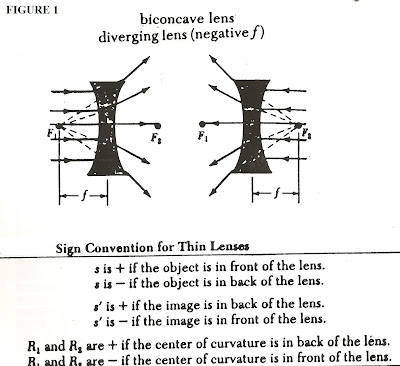## Monday, June 20, 2011

### Introduction to Basic Physics (Thin Lenses) - Pt. 19We now conclude our examination of single refracting surfaces as thin lenses, with a look at the diverging lens. In the previous segments-instalments we focused on converging lens, namely the biconvex lens, and now we focus on the biconcave lens.

Figure 1 shows the basic directions of the diverging rays from foci (F1, F2) of a single biconcave lens. The key point embodied in the diagram is that the focal length (f) is negative. This must be factored into all lens computations (such as when one is asked to find the image and-or object distances) for such lenses.

The bottom of Fig. 1 summarizes all the sign conventions for use with both converging and diverging thin lenses. It shows how the quantities s, s', R1 and R2 all change their sign in respect of where object or image is placed in reference to the lens, or where the centers of curvature are placed.

Figure 2 shows a typical ray construction as it would be applied to a diverging lens and in many ways is similar to the convergins lens except that now the object is formed on the same side of the lens as the image and is larger than the image. To summarize the numbered rays:

(1) Is drawn from the top of the object (O) to the optical axis of the lens.

(2) Is drawn from the top of O through the center of the lens and continued as a straight line

(3) Is drawn from the top of I (image) to the lens optical axis, thence to the oppostie focus (F)

(4) is drawn starting from the first focus (F) on the left side of the lens, intesecting the top of I then on toward the optical axis at upper part of lens.

We designate (1)- (3) as Principal Rays and (4) as an ancillary or adjunct ray.

Sample Problem:

The focal length of a diverging lens is 30 cm, and the object is 40 cm away. Find the image distance.

We have: f = - 30 cm

s = 40 cm

The thin lens equation for diverging lenses is:

1/s + 1/ s' = - 1/f

and in this case, we will write:

1/40 + 1/ s' = - 1/30

Then:

1/s' = -1/f -1/s = - 1/30 - 1/40

1/s' = - 7/120

s' = -120/7 cm = -17.1 cm

According to our sign conventions (Fig. 1, bottom) s' is negative if the image is in front of the lens. Since s' = -17.1 cm it must be located 17.1 cm in front of th elens, conforming with what we see in the ray diagram of Fig. 2

1. Using a graphical construction find the image formed by a diverging lens with a focal length of 30 cm when the object is 60 cm from the lens.

2. A diverging lens has a focal length of 20 cm.

(a) Where is the image if the object is 20 cm from the lens?

(b) If the object is 4 cm high, how high is the image? (Recall the lateral magnification of a thin lens - with sign conventions in place is:

M = h'/ h = -s'/s

3. A diverging lens placed 10 cm from an obnect produces an image which is half the size of the object. Find the focal length of the lens.